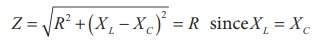Home | | Physics 12th Std | Resonance in series RLC Circuit

# Resonance in series RLC Circuit

The circuit is said to be in electrical resonance. The frequency at which resonance takes place is called resonant frequency.

Resonance in series RLC Circuit

When the frequency of the applied alternating source ( Žēr ) is equal to the natural  frequency | 1/ ŌłÜ(LC) | of  the  RLC circuit, the current in the circuit reaches its maximum value. Then the circuit is said to be in electrical resonance. The frequency at which resonance takes place is called resonant frequency.

Resonant angular frequency, Žēr = 1/ ŌłÜ(LC)Since XL and XC are frequency dependent, the resonance condition ( X L = XC ) can be achieved by the varying the frequency of the applied voltage.## Effects of series resonance

When series resonance occurs, the impedance of the circuit is minimum and is equal to the resistance of the circuit. As a result of this, the current in the circuit becomes maximum. This is shown in the resonance curve drawn between current and frequency (Figure 4.54).

At resonance, the impedance isTherefore, the current in the circuit isThe maximum current at series resonance is limited by the resistance of the circuit. For smaller resistance, larger current with sharper curve is obtained and vice versa.

## Applications of series RLC resonant circuit

RLC circuits have many applications like filter circuits, oscillators, voltage multipliers etc. An important use of series RLC resonant circuits is in the tuning circuits of radio and TV systems. The signals from many broadcasting stations at different frequencies are available in the air. To receive the signal of a particular station, tuning is done.

The tuning is commonly achieved by varying capacitance of a parallel plate variable capacitor, thereby changing the resonant frequency of the circuit. When resonant frequency is nearly equal to the frequency of the signal of the particular station, the amplitude of the current in the circuit is maximum. Thus the signal of that station alone is received.

Tags : Phasor diagram, Circuit Diagram, Formula | Alternating Current (AC) , 12th Physics : Electromagnetic Induction and Alternating Current
Study Material, Lecturing Notes, Assignment, Reference, Wiki description explanation, brief detail
12th Physics : Electromagnetic Induction and Alternating Current : Resonance in series RLC Circuit | Phasor diagram, Circuit Diagram, Formula | Alternating Current (AC)